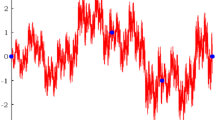# Riemann–Liouville calculus on quadratic fractal interpolation function with variable scaling factors

## Abstract

In this paper, we explore the Riemann–Liouvllie fractional calculus of quadratic fractal interpolation function (QFIF) with variable scaling factors. Fractional calculus of QFIF with predefined initial condition is investigated in an arbitrary closed interval of $$\mathbb {R}$$. Further, the relation between the order of fractional integral (derivative) and the box dimension of QFIF is established.

This is a preview of subscription content, log in via an institution to check access.

Price excludes VAT (USA)
Tax calculation will be finalised during checkout.

### Similar content being viewed by others### On the variable order fractional calculus of fractal interpolation functions

R. Valarmathi & A. Gowrisankar### Riemann–Liouville fractional integral of non-affine fractal interpolation function and its fractional operator

T. M. C. Priyanka & A. Gowrisankar### Variable order fractional calculus on $$\alpha$$ -fractal functions

R. Valarmathi & A. Gowrisankar

## References

1. Barnsley, M.F. 1986. Fractal functions and interpolation. Constructive Approximation 2 (1): 303–329.

2. Barnsley, M.F., and A.N. Harrington. 1989. The calculus of fractal interpolation functions. Journal of Approximation Theory 57 (1): 14–34.

3. Tatom, F.B. 1995. The relationship between fractional calculus and fractal. Fractals 3 (1): 217–229.

4. Yao, K., W.Y. Su, and S.P. Zhou. 2005. On the connection between the order of fractional calculus and the dimensions of a fractal function. Chaos, Solitons & Fractals 23: 621–629.

5. Ruan, Huo-Jun, Su Wei-Yi, and Kui Yao. 2009. Box dimension and fractional integral of linear fractal interpolation functions. Journal of Approximation Theory 161: 187–197.

6. Liang, Y.S., and W.Y. Su. 2016. Fractal dimensions of fractional integral of continuous functions. Acta Mathematica Sinica 32 (12): 1494–1508.

7. Gowrisankar, A., and R. Uthayakumar. 2016. Fractional calculus on fractal interpolation function for a sequence of data with countable iterated function system. Mediterranean Journal of Mathematics 13 (6): 3887–3906.

8. Xiao, E.W., and H.D. Jun. 2017. Box dimension of Hadamard fractional integral of continuous functions of bounded and unbounded variation. Fractals 25 (3): 1750035.

9. Li, Y., and Y. Liang. 2017. Upper bound estimation of fractal dimension of fractional calculus of continuous functions. Advances in Analysis 2 (2): 121–128.

10. Liang, Y.S., and Q. Zhang. 2016. A type of fractal interpolation functions and their fractional calculus. Fractals 24 (2): 1650026.

11. Diethelm, Kai. 2010. The analysis of fractional differential equations. Berlin: Springer.

12. Kilbas, A.A., H.M. Srivastava, and J.J. Trujillo. 2006. Theory and applications of fractional differential equations. Sydney: Elsevier.

13. Wang, H.Y., and J.S. Yu. 2013. Fractal interpolation functions with variable parameters and their analytical properties. Journal of Approximation Theory 175: 1–8.

14. Navascues, M.A. 2006. A fractal approximation to periodicity. Fractals 14 (4): 315–325.

15. Chand, A.K.B., and N. Vijender. 2014. Monotonicity preserving rational quadratic fractal interpolation functions. Advances in Numerical Analysis 504825: 17.

16. Chand, A.K.B., M.A. Navascues, P. Viswanathan, and S.K. Katiyar. 2016. Fractal trigonometric polynomials for restricted range approximation. Fractals 24 (2): 1650022.

## Author information

Authors

### Corresponding author

Correspondence to A. Gowrisankar.

## Ethics declarations

### Conflict of interest

The authors have declared that no conflict of interest exists.

### Informed consent

The research does not involve any human participants or animals. Therefore, we do not need the statement on welfare of human participants or animals.